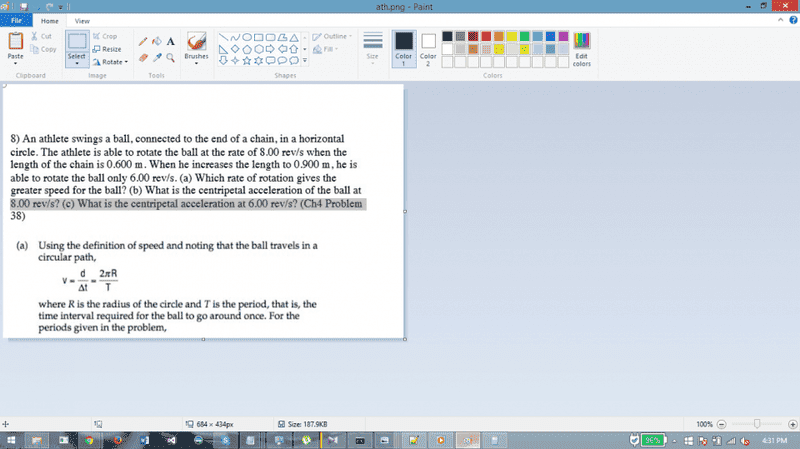# Why is ##2 \pi /T## multiplied by R for v?

Hey all, $$v = 2 \pi f =2 \pi \frac{1}{T} =\frac{2 \pi }{T}$$ but why is it multiplied by $$R$$? Any help appreciated.I see now why R is multiplied in now, but why inst L multiplied in in the analogous pendulum equation?

vanhees71
Gold Member
2021 Award
A particle moving with constant angular velocity ##\omega## on a circle is decribed by the position vector
$$\vec{x}=\begin{pmatrix} r \cos(\omega t) \\ r \sin (\omega t) \\ 0 \end{pmatrix}.$$
The time derivative gives the velocity
$$\dot{\vec{x}}=\vec{v}=\begin{pmatrix} -r \omega \sin(\omega t) \\ r \omega \cos(\omega t) \end{pmatrix}.$$
The magnitude thus is
$$|\vec{v}|=r \omega.$$

•Superposed_Cat and QuantumQuest
QuantumQuest
Gold Member
The first relation you write is angular frequency not velocity: ##\omega = \frac{2\pi}{T}##. Then ##\upsilon = \frac{2\pi}{T} R = \omega R##

•Superposed_Cat
vanhees71